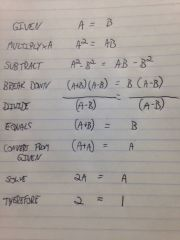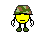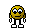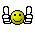Guest views are now limited to 12 pages. If you get an "Error" message, just sign in! If you need to create an account, click here.

• 1
17
• 9
14

## Recommended Posts•1

1

9

9

##### Share on other sites

1##### Share on other sites
So, long ago people agreed to follow rules when doing calculations, and they are:

Order of Operations

Do things in Parentheses First. Example:

yes 6 × (5 + 3) = 6 × 8 = 48

no 6 × (5 + 3) = 30 + 3 = 33 (wrong)

Exponents (Powers, Roots) before Multiply, Divide, Add or Subtract. Example:

yes 5 × 22 = 5 × 4 = 20

no 5 × 22 = 102 = 100 (wrong)

Multiply or Divide before you Add or Subtract. Example:

yes 2 + 5 × 3 = 2 + 15 = 17

no 2 + 5 × 3 = 7 × 3 = 21 (wrong)

Otherwise just go left to right. Example:

yes 30 ÷ 5 × 3 = 6 × 3 = 18

no 30 ÷ 5 × 3 = 30 ÷ 15 = 2 (wrong)

How Do I Remember It All ... ? PEMDAS !

P Parentheses first

E Exponents (ie Powers and Square Roots, etc.)

MD Multiplication and Division (left-to-right)

Divide and Multiply rank equally (and go left to right).

Add and Subtract rank equally (and go left to right)

Oh, yes, and what about 7 + (6 × 52 + 3) ?

7 + (6 × 52 + 3)

7 + (6 × 25 + 3) Start inside Parentheses, and then use Exponents First

7 + (150 + 3) Then Multiply

7 + 153 Parentheses completed, last operation is an Add

160 DONE !

its 9

##### Share on other sites

that used to be easy! 9

Edited by moose 57

##### Share on other sites

The answer is 1.  First solve in the (2+1) = 3.  Then multiply 3 X 2 = 6.  Then divide 6 / 6 = 1.

Try this one.  My 9th grade algebra teacher, Mr. Wiley would always put up a brain teaser and leave it on the board until we figured it out.  He was a great teacher, the kind that taught you not just algebra but how to think.  This one stayed on the board until the end of the semester.  I have several teacher friends that have taken it to their math departments and almost no one can figure it out.  I give you for your ciphering pleasure 2 = 1.

##### Share on other sites

The answer is 1.  First solve in the (2+1) = 3.  Then multiply 3 X 2 = 6.  Then divide 6 / 6 = 1.

Try this one.  My 9th grade algebra teacher, Mr. Wiley would always put up a brain teaser and leave it on the board until we figured it out.  He was a great teacher, the kind that taught you not just algebra but how to think.  This one stayed on the board until the end of the semester.  I have several teacher friends that have taken it to their math departments and almost no one can figure it out.  I give you for your ciphering pleasure 2 = 1.

Actually, the answer is nine (9).

You calculate left to right:

6 ÷ 2(1+2) = 1 or 9

3 (2+1) = 9

3 (3) = 9

.

##### Share on other sites

I stand corrected. I was mistakenly thinking of the division sign as an equal sign and solving both sides of the equation. Good one, but can you find the flaw in 2=1?

•1

##### Share on other sites
I stand corrected. After seeing this example " 30 ÷ 5 × 3 = 6 × 3 = 18"

I see where I was flawed in my thinking. I am not a math wiz but I know how to.

##### Share on other sites

I was taught Please Excuse My Dear Aunt Sally to help remember the calculation order.

##### Share on other sites

Scares me how easily we forget some of the basics.

##### Share on other sitesI stand corrected. I was mistakenly thinking of the division sign as an equal sign and solving both sides of the equation. Good one, but can you find the flaw in 2=1?

When you start breaking it down, you end up with 0 = 0 and you're dividing by 0, which you can't do.•1

##### Share on other sites

I am and have been a Algebra 1 and Pre-Algebra teacher for the past 26 years. Those of you who have said that you solve what is inside the parentheses first ( 1 + 2 ) equaling 3 are correct. This is because parentheses is the highest priority and must be done first. I

Where the majority of people fail is where they believe that multiplication has to be done next because they are following the Please Excuse My Dear Aunt Sally protocol or PEMDAS. This is where there is a misunderstanding.

Multiplication and division are on the same priority level of importance in terms of when we finally get to use these skills The other rule then that has to used is to answer the question, "which math operation is on the left hand side of the remaining equation?"

The remaining equation is: 6 / 2 ( 3 ). So we must divide 6 by 2 which equals 3. Finally we perform the last math operation of multiplication and 3 ( 3 ) = 9. I hope this helps.

When I teach these concepts, I always show the math with a written explanation as to why we took a specific action. I don't want to create robots but thinking people who know why a certain action was required at a given point within an equation. Even when I have my stronger students tutoring other classmates, I always make sure I hear them ask the other student, "why did you solve this part of the equation next?" I tell them to wait until they are given an explanation before moving on.

•4

##### Share on other sites

When you start breaking it down, you end up with 0 = 0 and you're dividing by 0, which you can't do.Winner winner chicken dinner, you are correct (and more observant than most of the math department at USD035).  What Mr. Wiley wanted to demonstrate is that you can add, subtract, and multiply with 0 and still have a correct answer but if you divide by 0 the only correct answer is “undefined”.

Thanks Mark, for the Friday night brain exercise.  It’s like anything else in that if you do not exercise the brain, atrophy will set in (and judging by current events cranial atrophy has already set in on much of the population).

•1

##### Share on other sites

the answer  is correct !      the sum is equal too the  equation ,  the  end values are  equal in amounts,  take the   equation and  divide them with each other and  it is  1

##### Share on other sites•3

##### Share on other sites

I choose 9 based on what I was taught...!An interesting equationThanks for posting...!

##### Share on other sites

I have a pretty good accountant and if I ask him this equation his answer would be  " what do you want it to equal?"

But Of course its 9  - Thanks Markinsa

•1

##### Share on other sites

2+1=3.....then 6/2= 3......then 3x3=9##### Share on other sites

My calculator says the answer is 1!!##### Share on other sites

This is not algebra, although you can solve it with algebra. It is a simple, 7th grade division problem.  Everyone is trying to make it so difficult and are getting confused.  It is the number 6 divided by the number 2(2+1). Now set it up as a division problem and solve.

6                         6                     6

----------- =        ------------=           ----=  1

2(2+1)                   4+2                   6

2(2+1) is one number and must be cleared before you divide into six.  I believe that is where the confusion has arisen.

##### Share on other sites

I/m on the WRONG page!

•1

##### Share on other sitesThis topic is now closed to further replies.